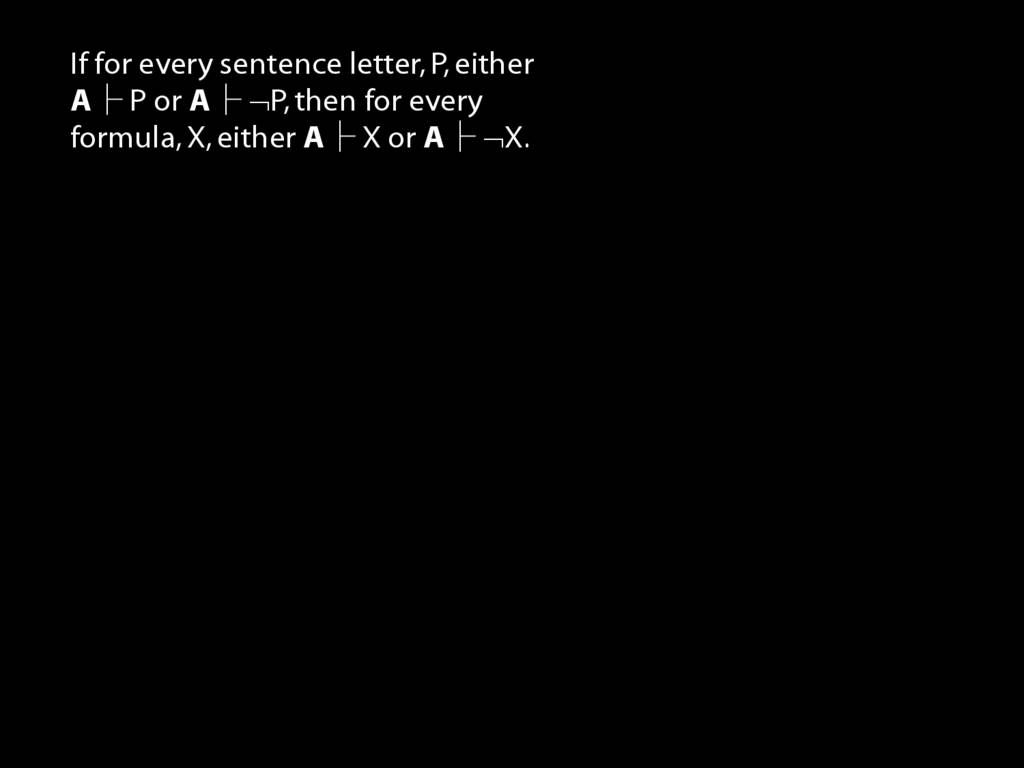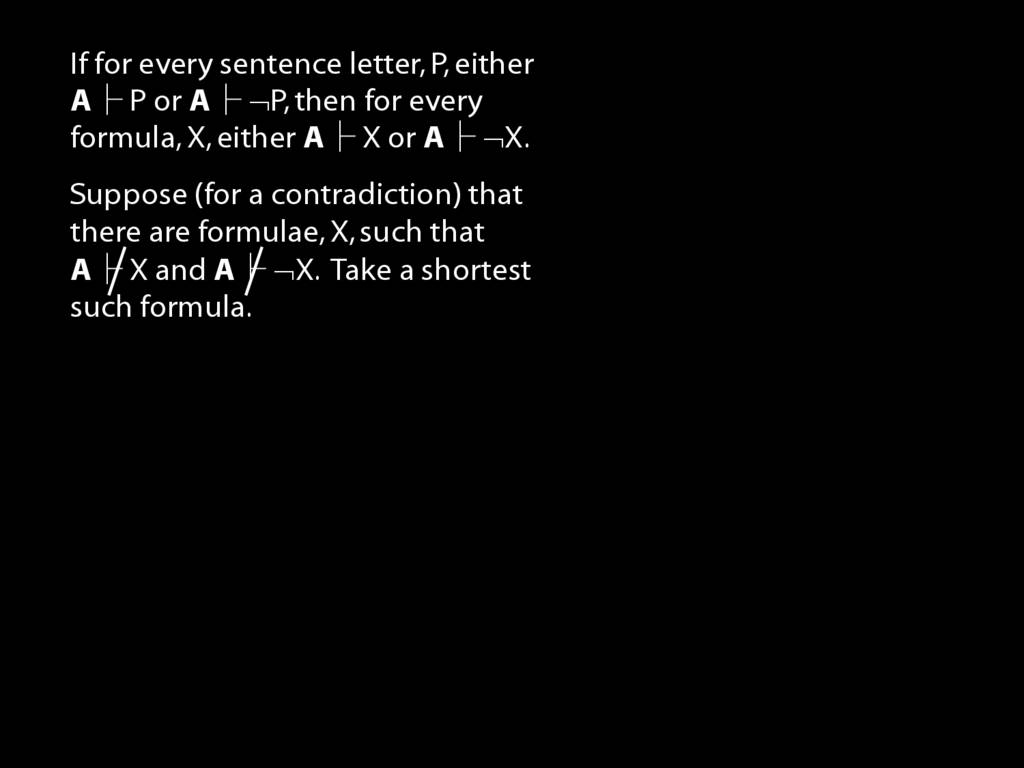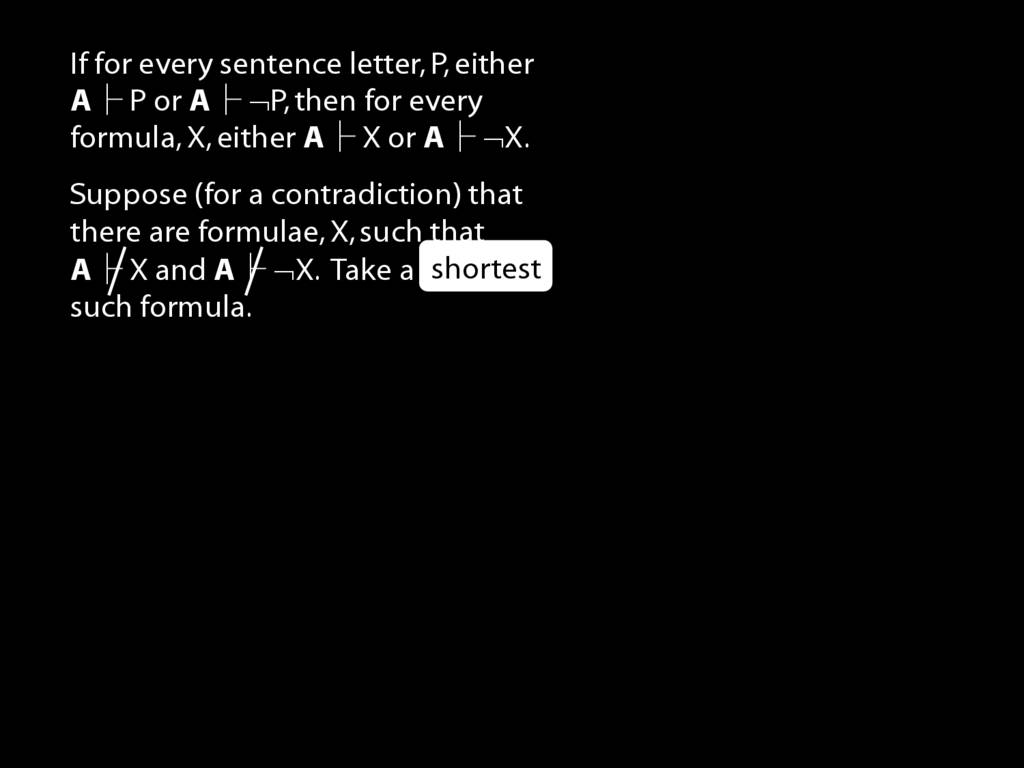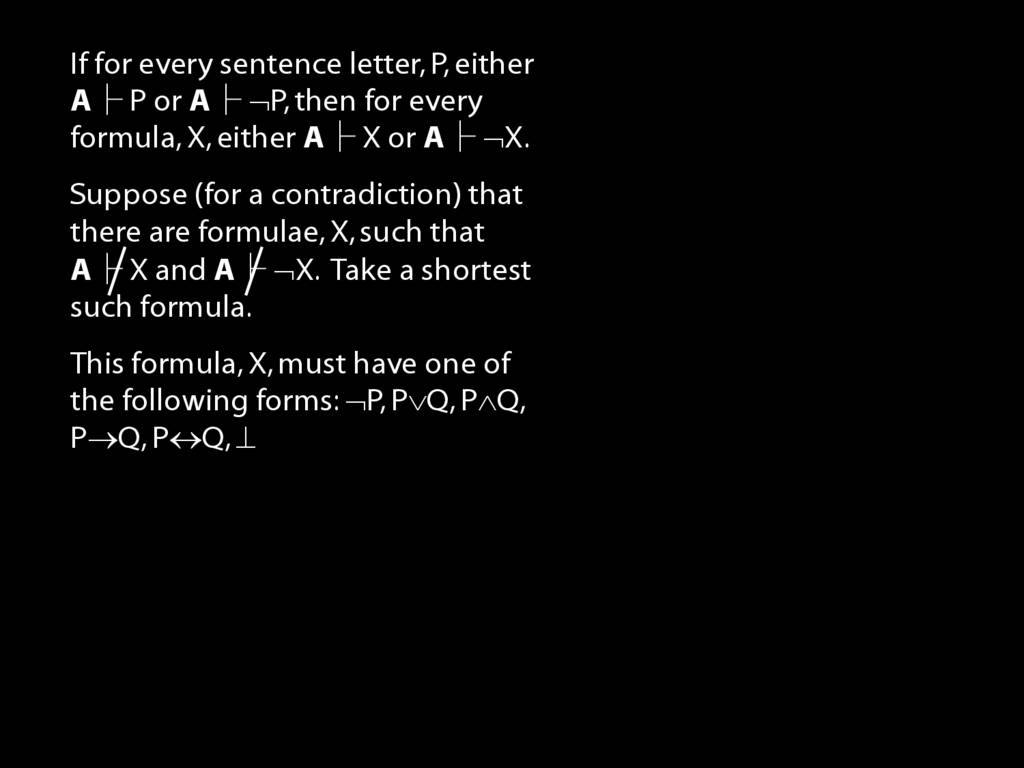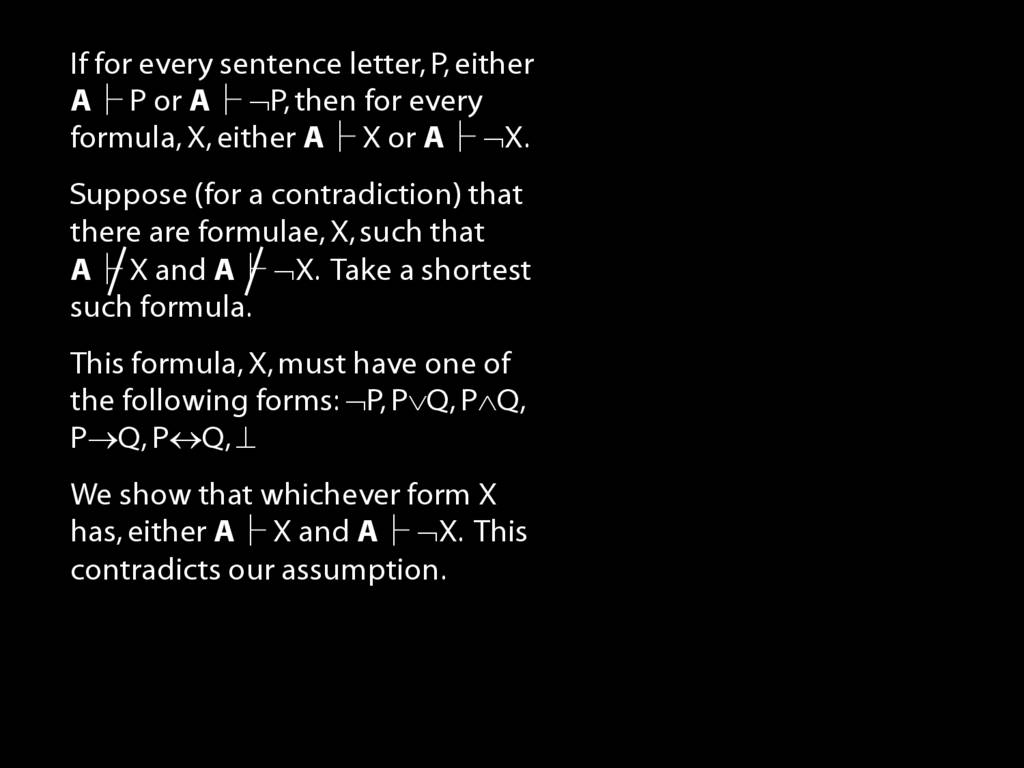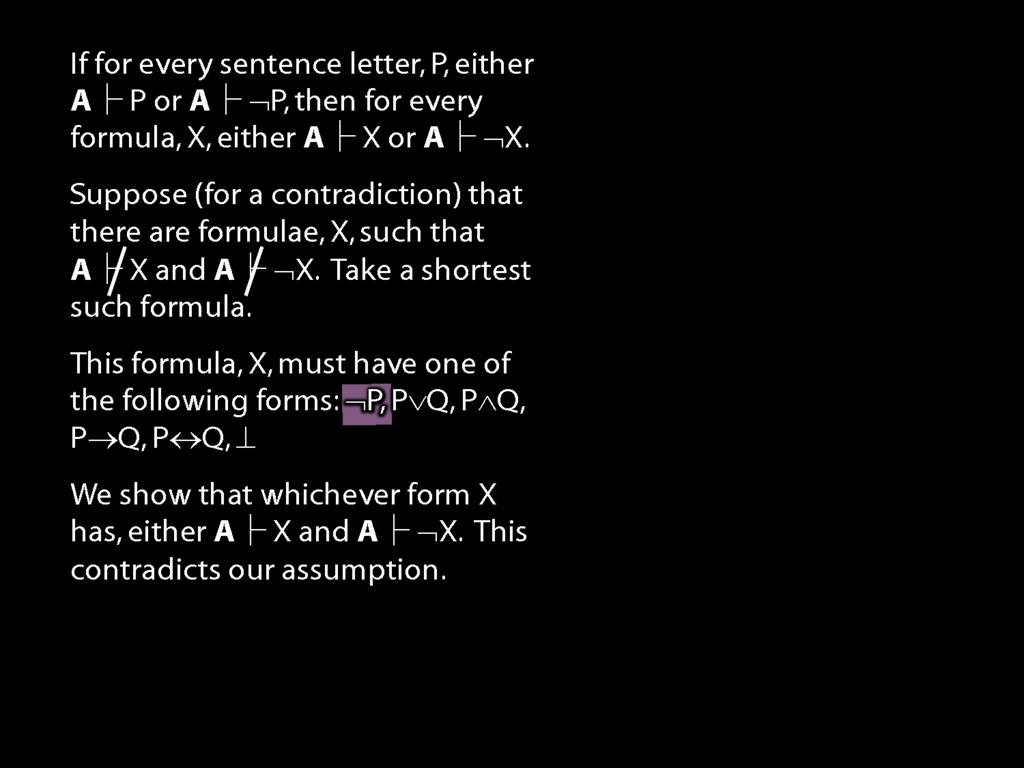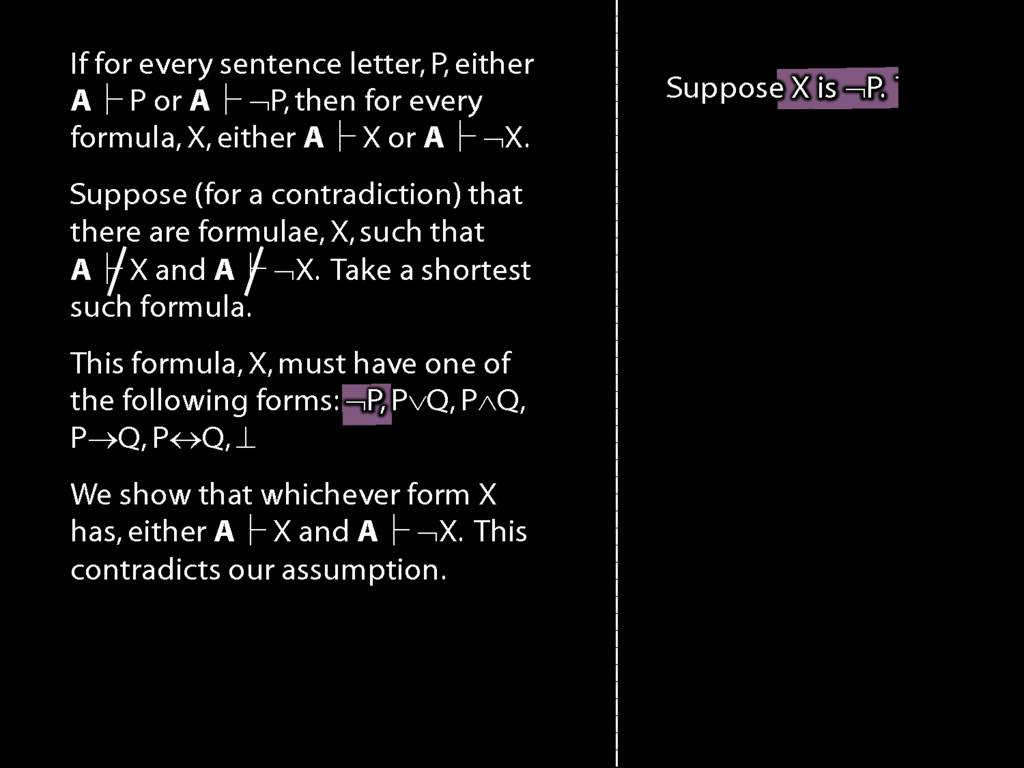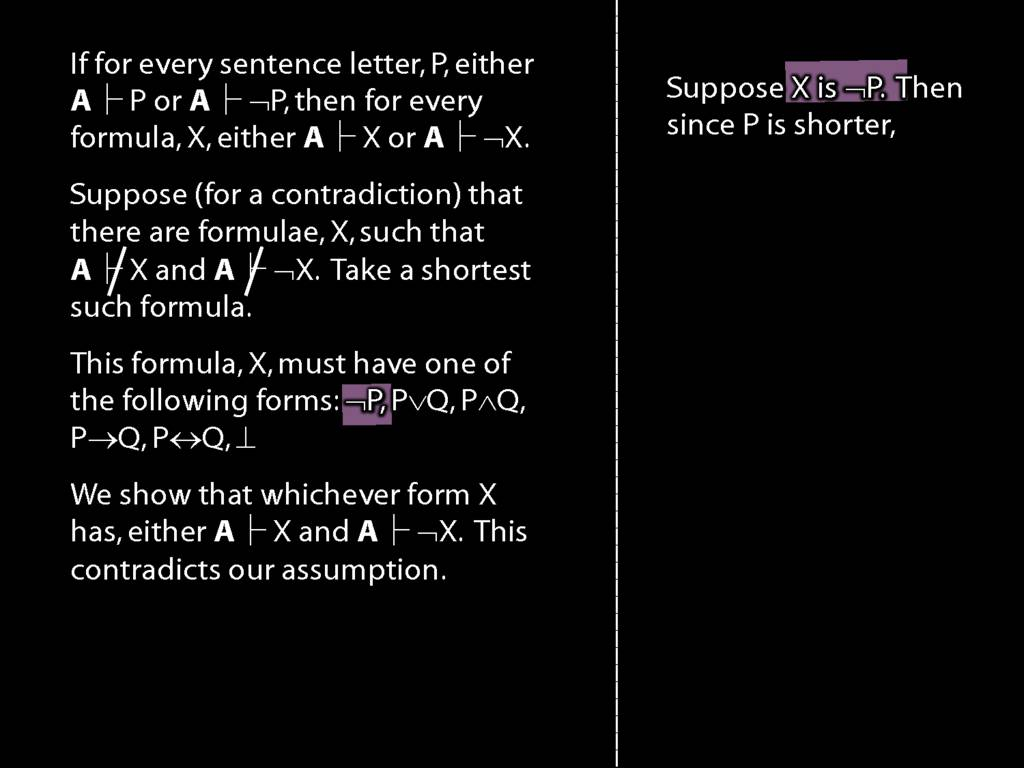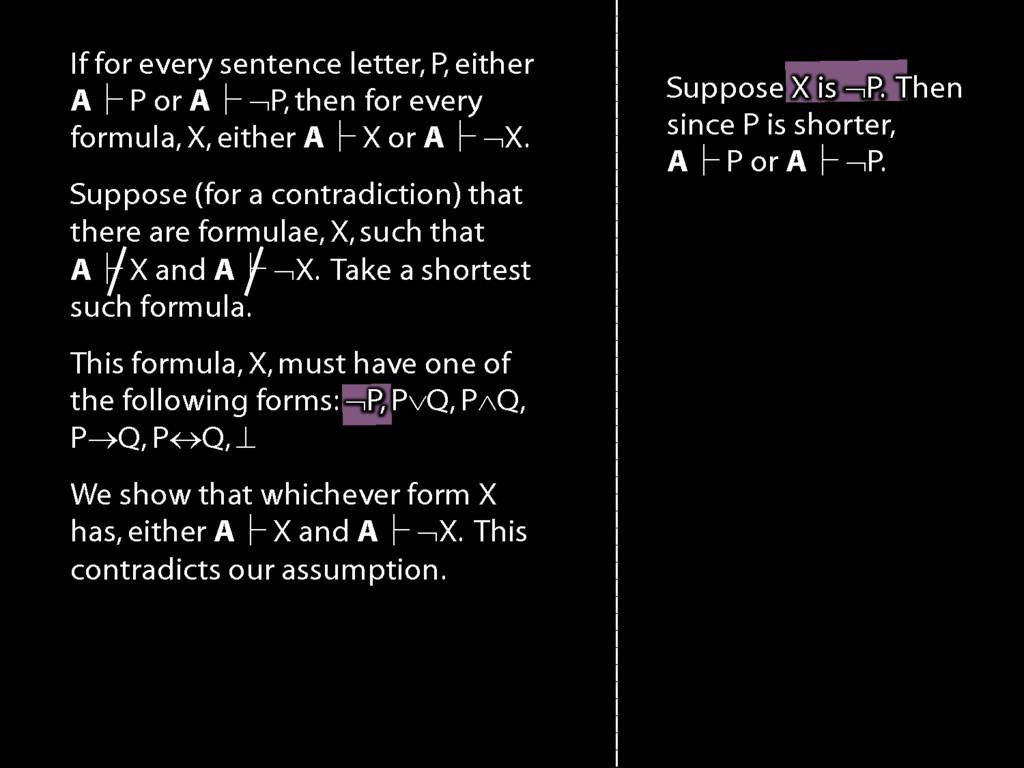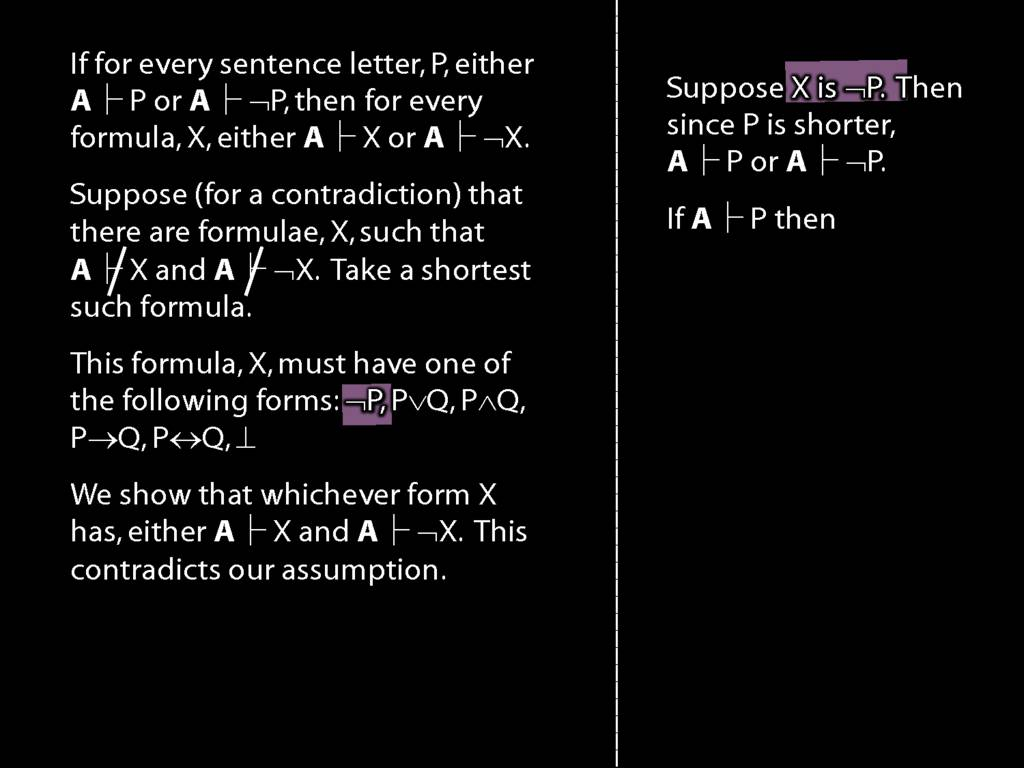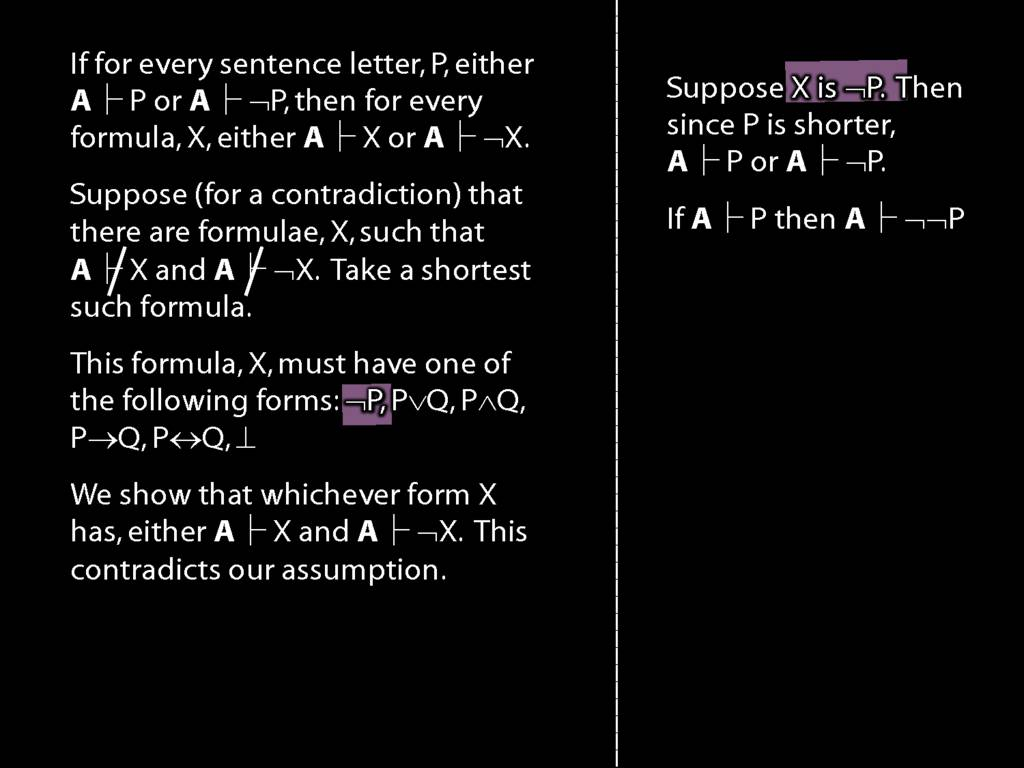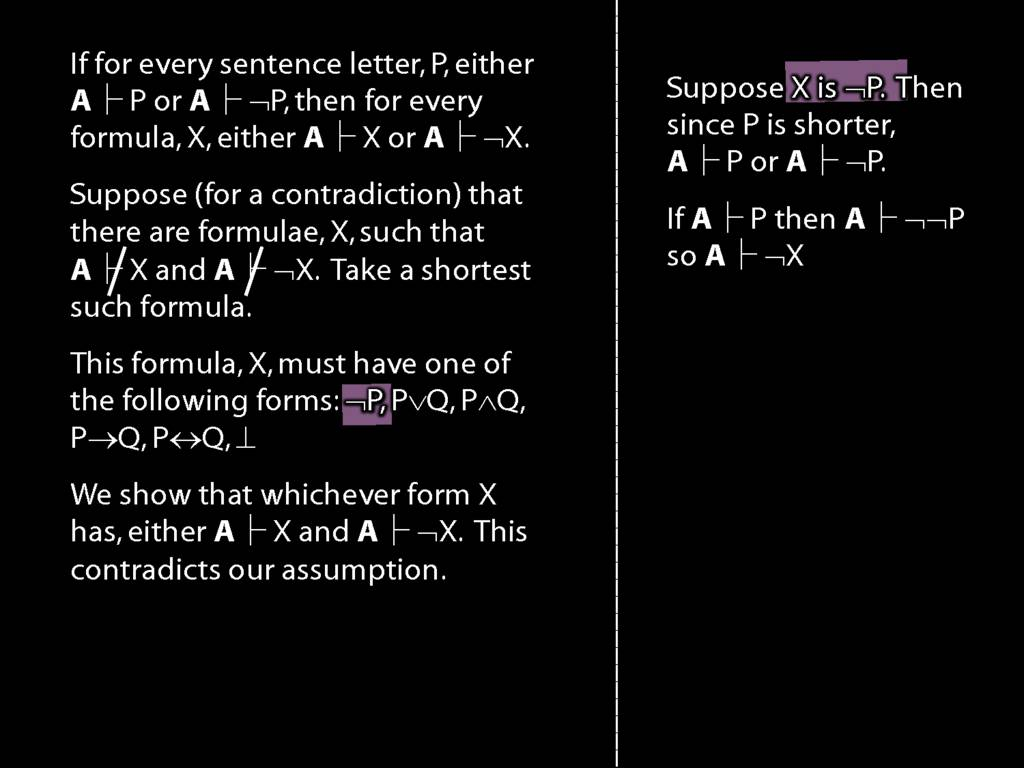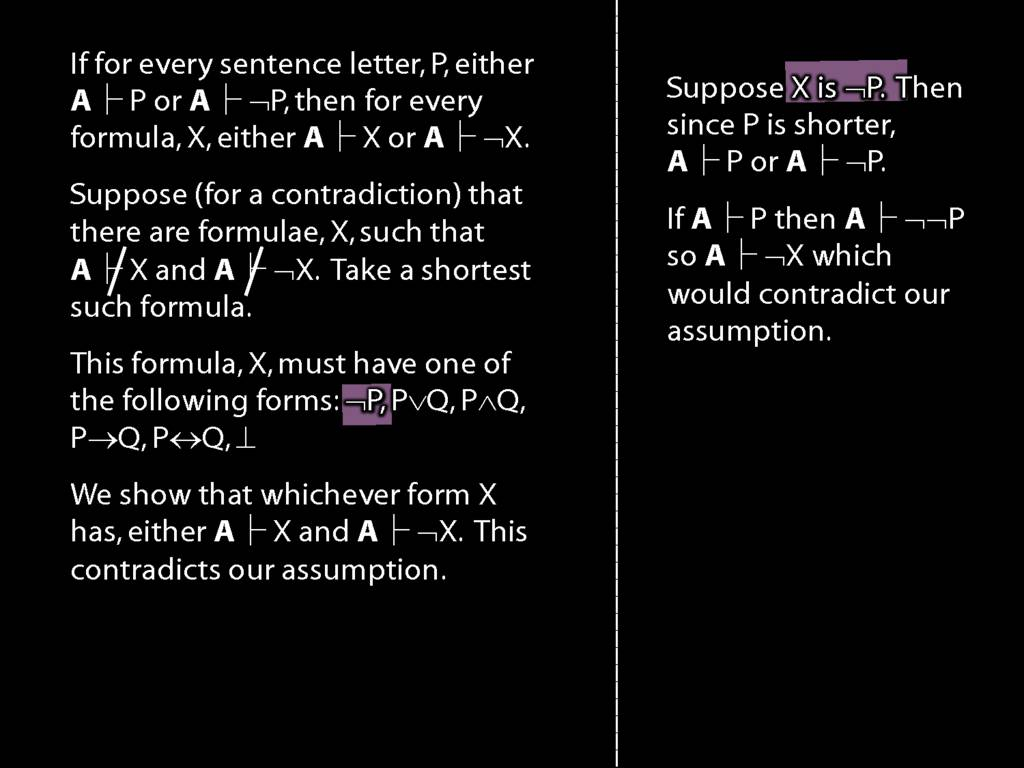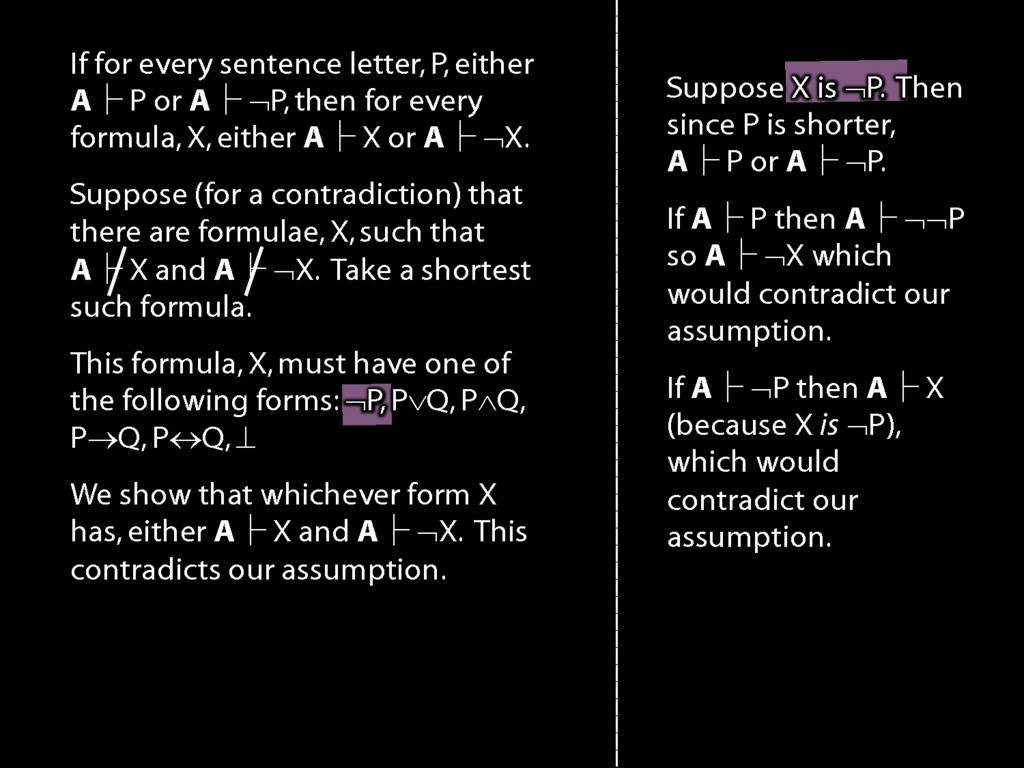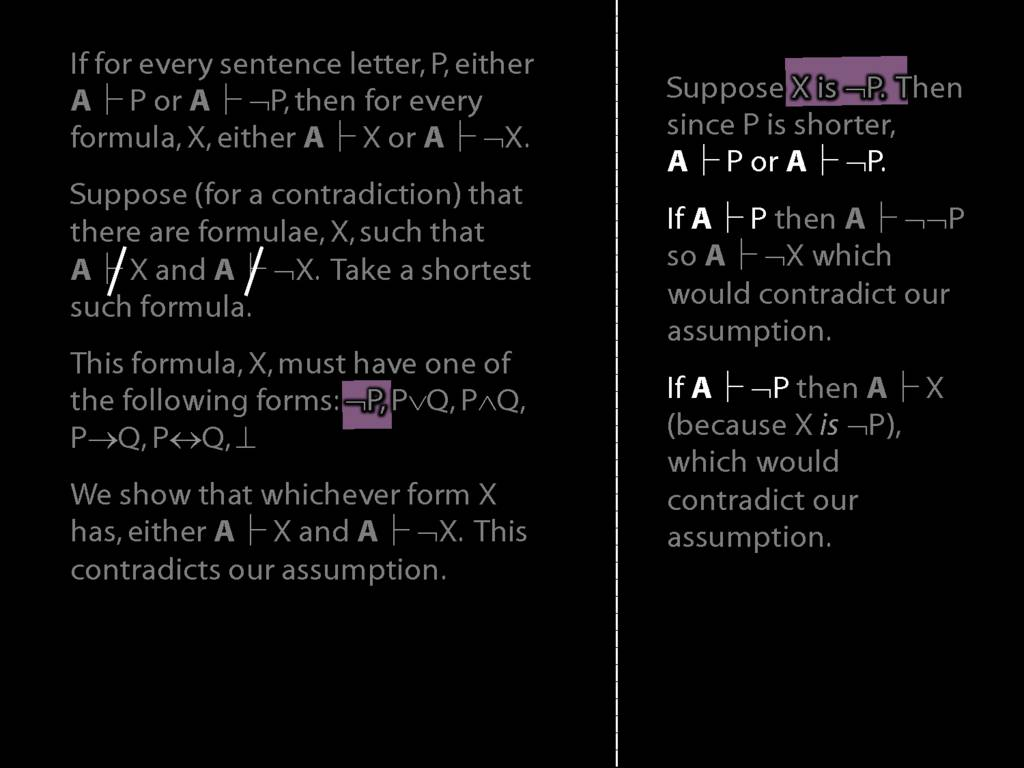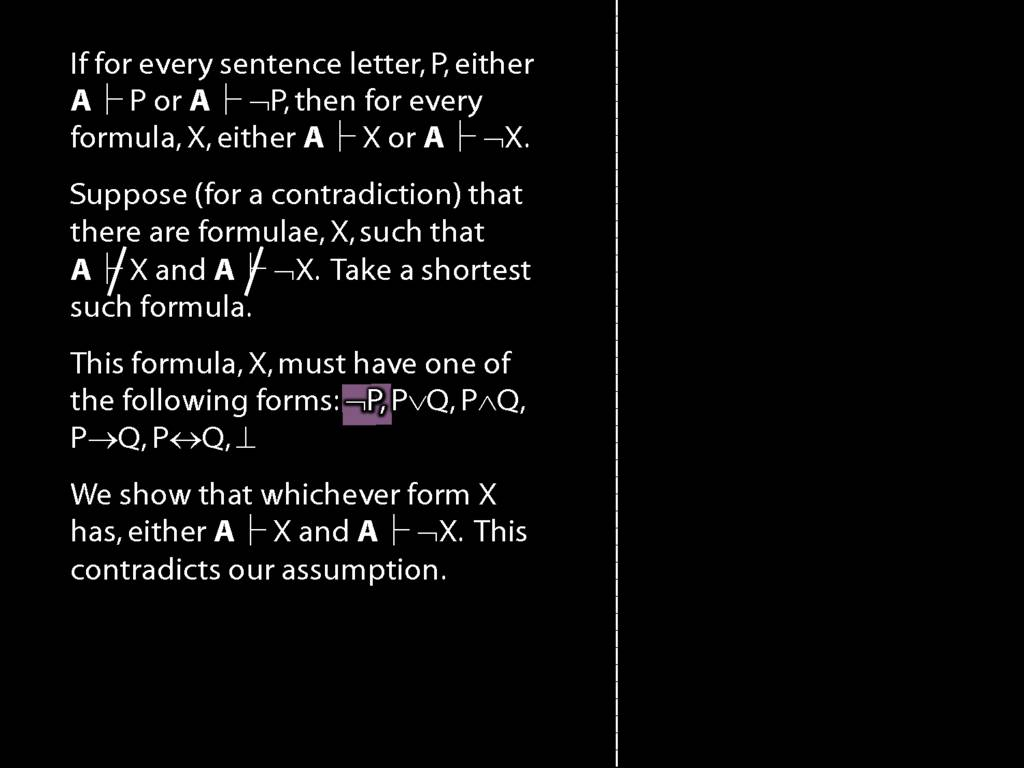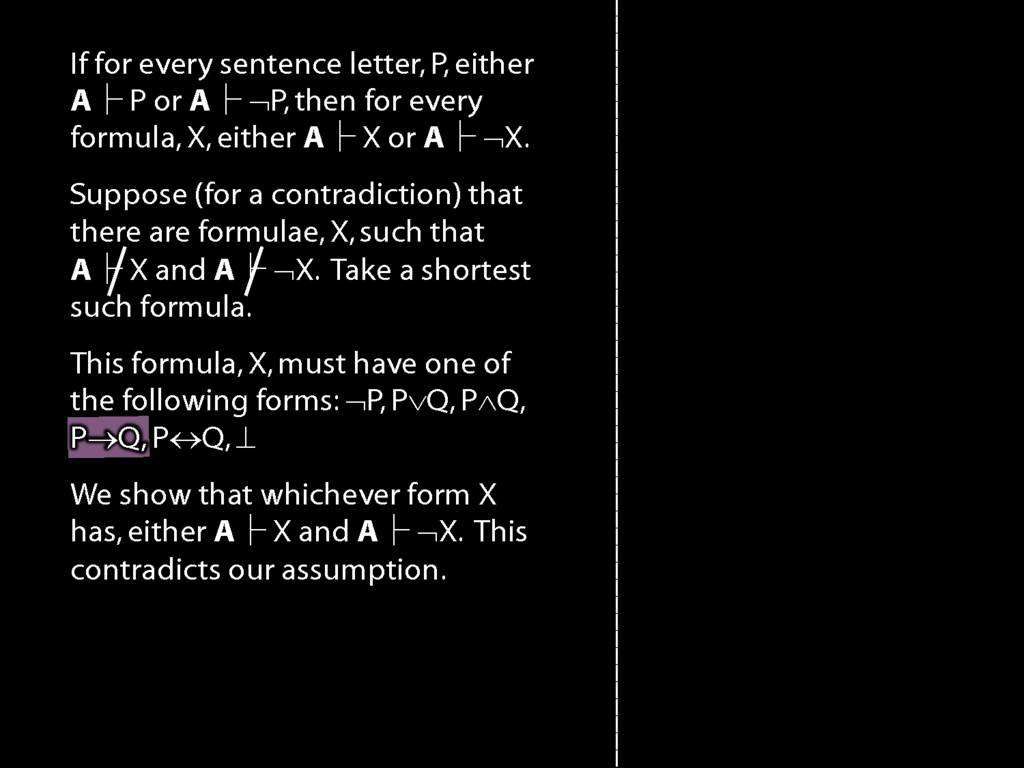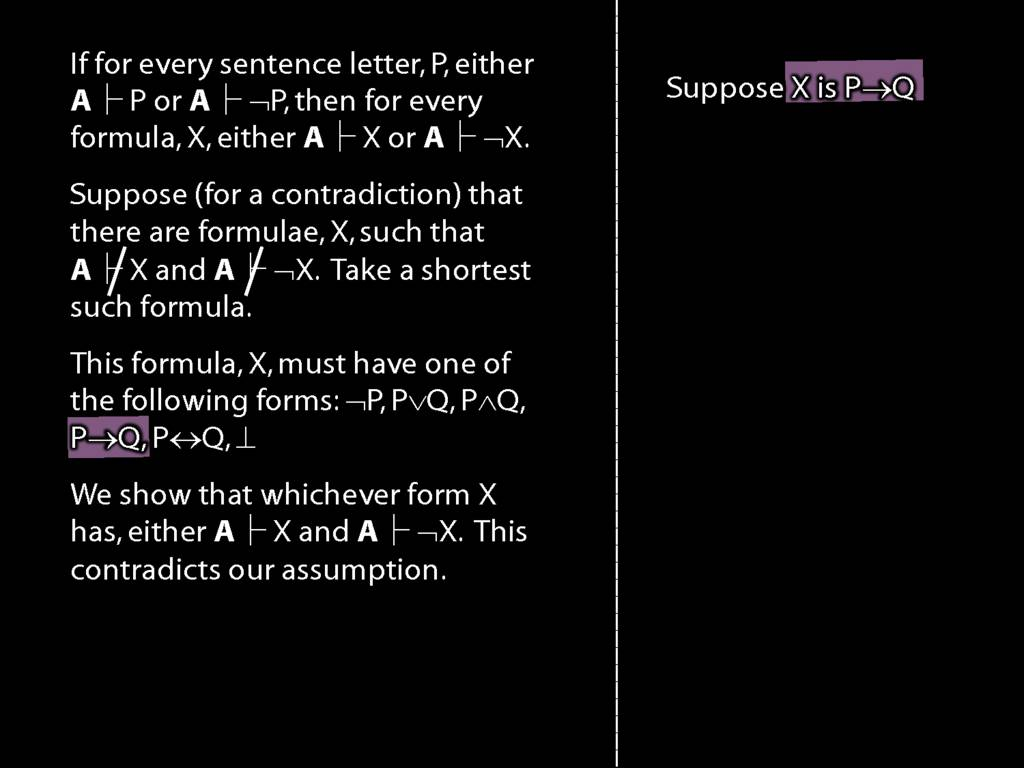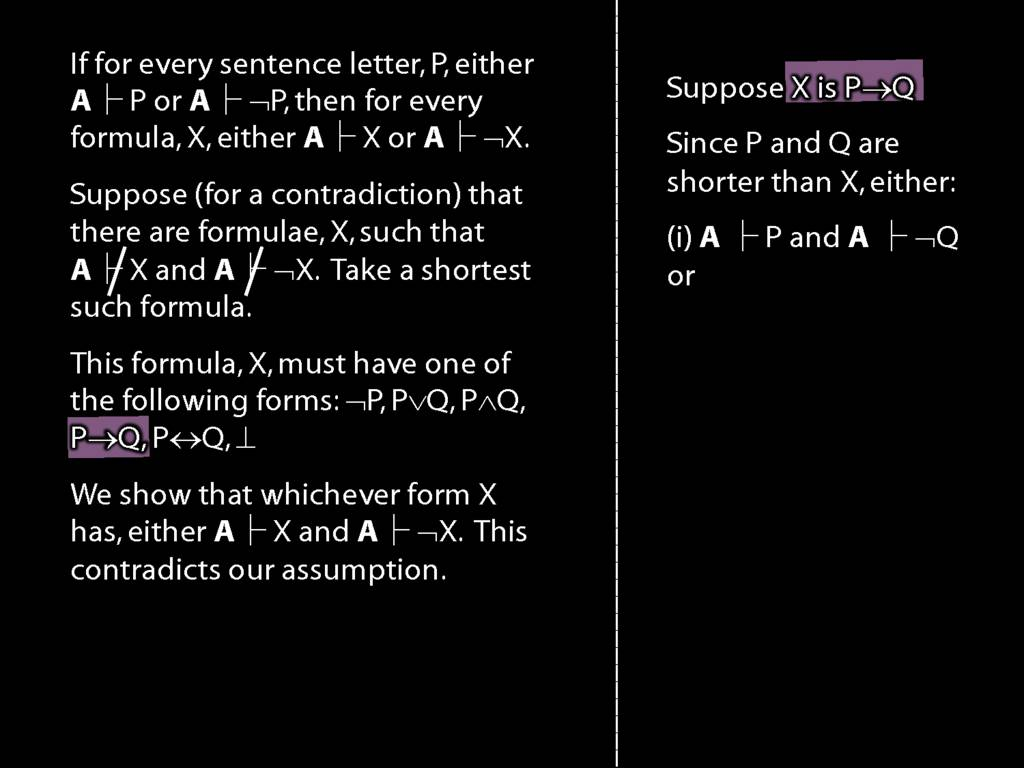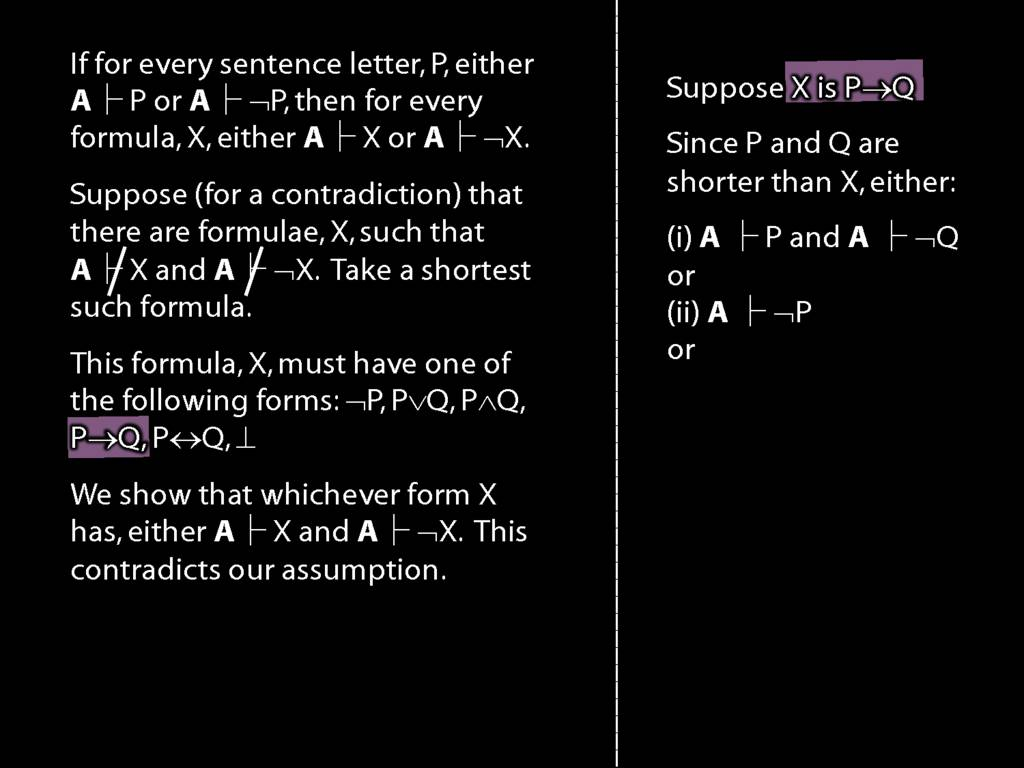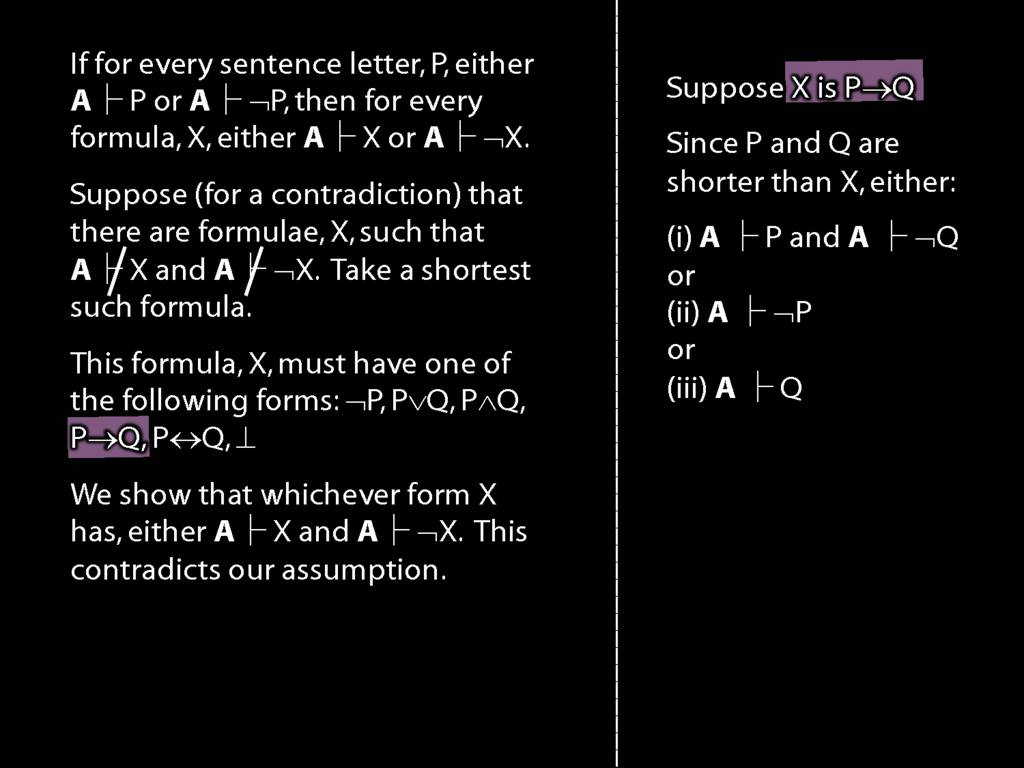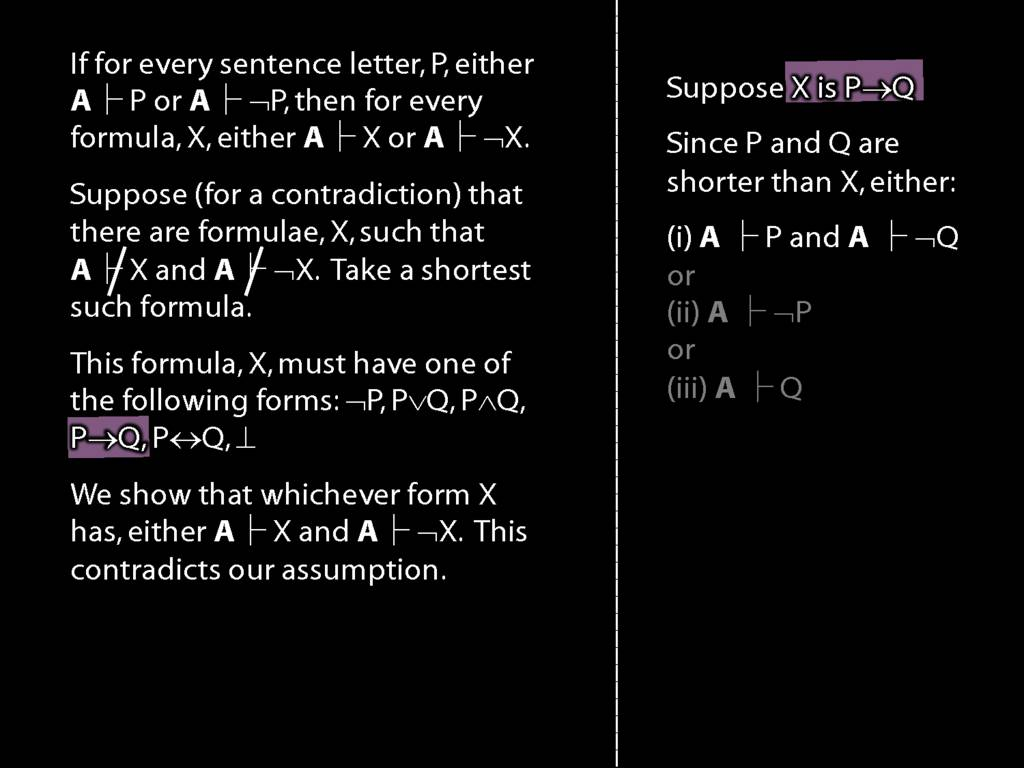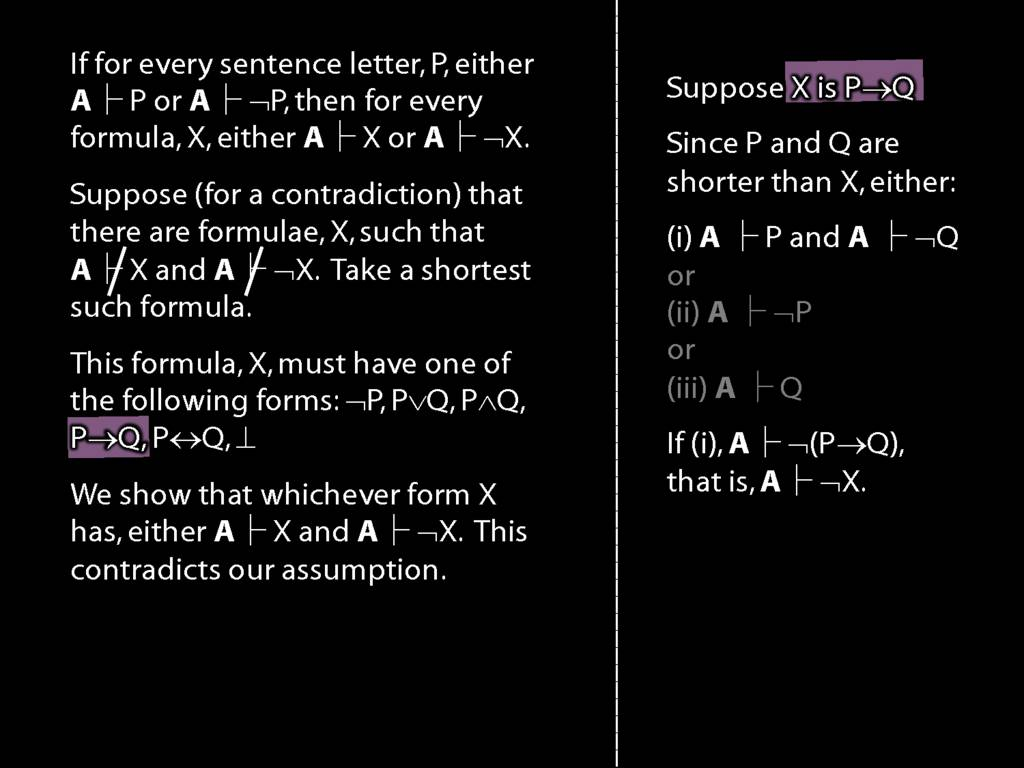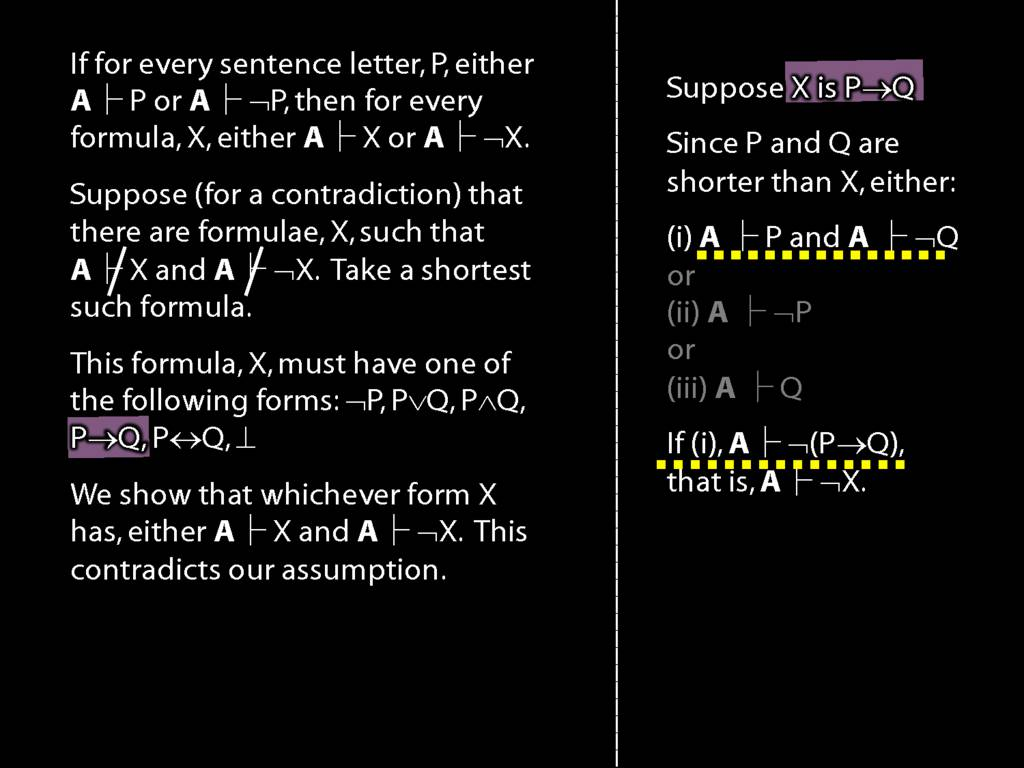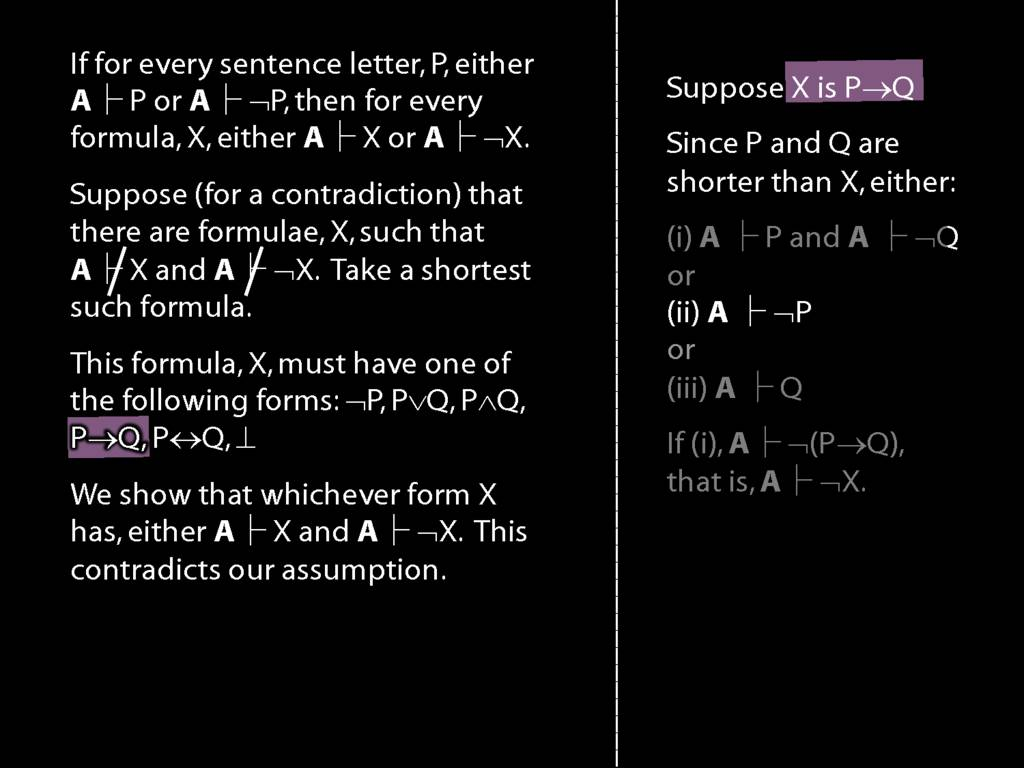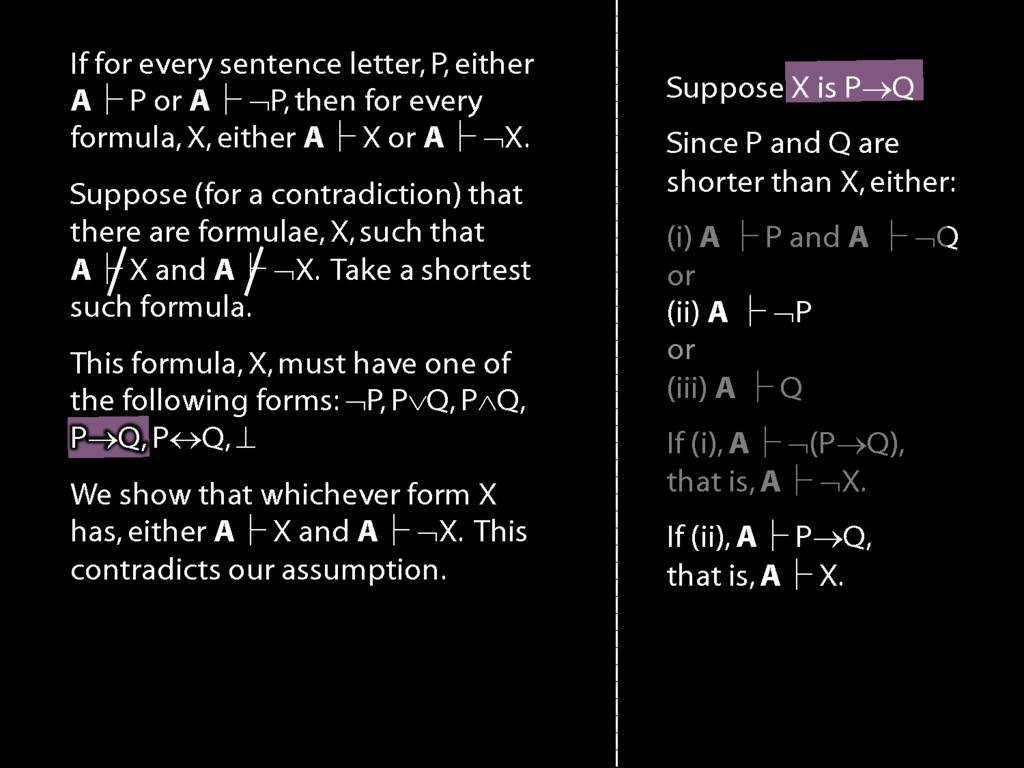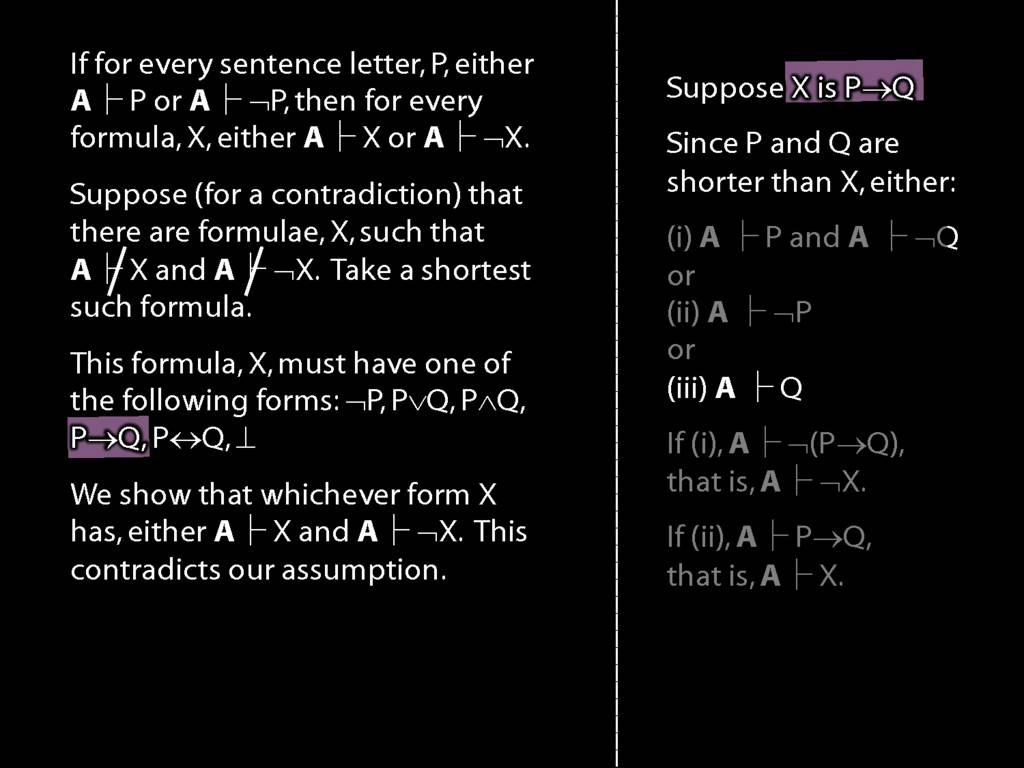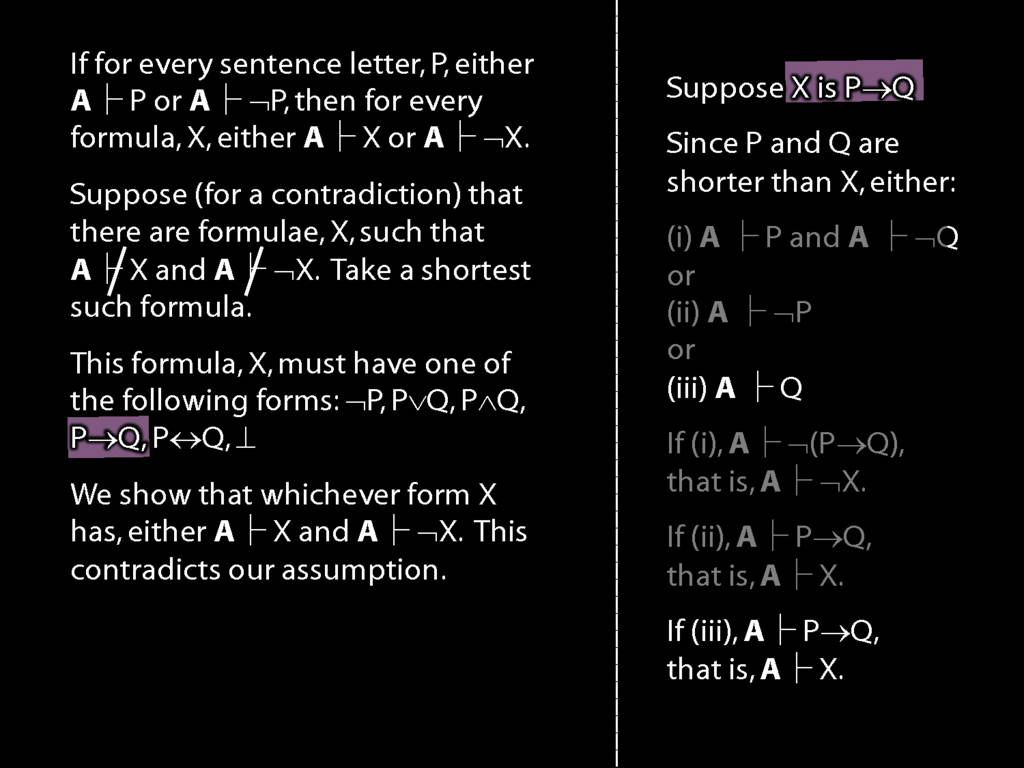Press the right key for the next slide (or swipe left)

also ...

Press the left key to go backwards (or swipe right)

Press n to toggle whether notes are shown (no equivalent if you don't have a keyboard)

Press m or double tap to see a menu of slides

## Lemma for the Completeness Theorem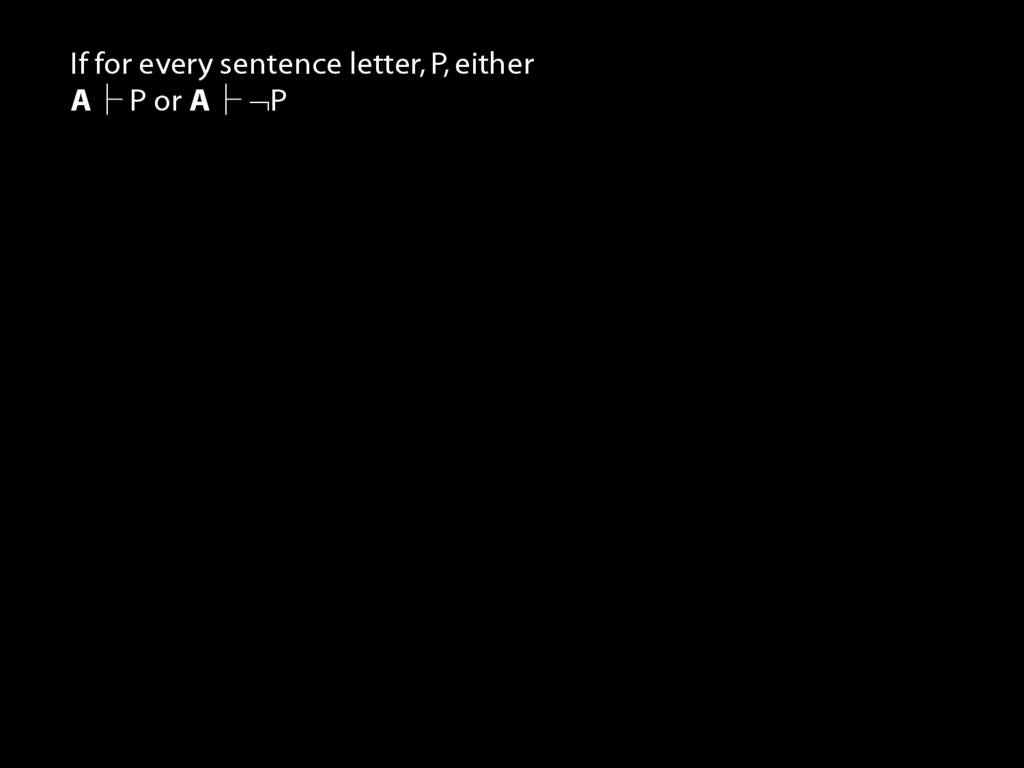If for every sentence letter, P, either A ⊢ P or A ⊢ ¬P, then for every formula, X, either A ⊢ X or A ⊢ ¬X.
Proof
\textbf{Step a.} Suppose (for a contradiction) that there are formulae, X, such that A ⊬ X and A ⊬ ¬X. Take a shortest such formula, call it Y.
\textbf{Step b.} This formula, Y, must have one of the following forms: ¬P, P∨Q, P∧Q, P→Q, P↔Q, ⊥
\textbf{Step c.} We can show that whichever form X has, either A ⊢ Y and A ⊢ ¬Y.
Case 1: X is P→Q. Then since P and Q are shorter than X, either:
\hspace{5mm} (i) A ⊢ P and A ⊢ ¬Q
\hspace{5mm} or
\hspace{5mm} (ii) A ⊢ ¬P
\hspace{5mm} or
\hspace{5mm} (iii) A ⊢ Q
\hspace{5mm} If (i), A ⊢ ¬(P→Q), that is, A ⊢ ¬X.
\hspace{5mm} If (ii), A ⊢ P→Q, that is, A ⊢ ¬X.
\hspace{5mm} If (iii), A ⊢ P→Q, that is, A ⊢ ¬X.
\hspace{5mm} (Here we use the last two Proofs about Proofs, see earlier)
Case 2: X is ¬P.
\hspace{5mm} Then since P is shorter, A ⊢ P or A ⊢ ¬P.
\hspace{5mm} If A ⊢ P then A ⊢ ¬¬P so A ⊢ ¬X which would contradict our assumption. This is shown in the proofs about proofs above.
\hspace{5mm} If A ⊢ ¬P then A ⊢ X (because X is ¬P), which would contradict our assumption.
Case 3: …
\textbf{Step d.} The demonstration in Step c contradicts our assumption, so we can conclude that it is false. That is, either A ⊢ X and A ⊢ ¬X for every formula X.